### Home > CALC > Chapter 6 > Lesson 6.1.3 > Problem6-38

6-38.
1. Differentiate the following functions with respect to the given independent variable. Homework Help ✎

1. y = 2log2 x

2. y = tan 10x

3. y = cos t tan t

4. y = tan(ex )

5. y = 2cos(w3)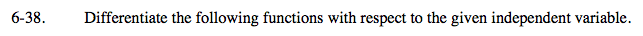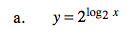2log2x = x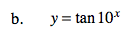Use the Chain Rule.

y' = (sec210x)(ln 10) · 10x

$\text{tan}x=\frac{\text{sin}x}{\text{cos}x}$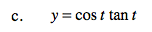$\text{Use the Chain Rule. }\frac{d}{dx}e^{x}=e^{x}$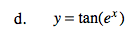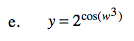y' = (ln 2) · 2cos(w3) · sin(w3) · 3w2